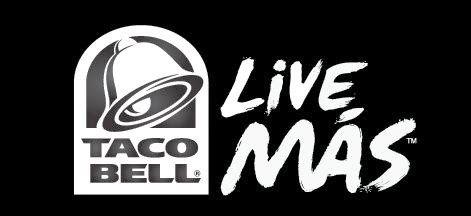## Saturday, April 26, 2014

### 33 Watch | KFC, Taco Bell, Pizza Hut

You might well have noticed that Kentucky Fried Chicken, Taco Bell, and Pizza Hut are all the same company.  Perhaps you've seen semi-trucks on the freeway baring all three stores symbols, or you've seen their specialty stores where all three restaurants are located at one site.  What is interesting about these three companies, is that they all have '33 numerology' and more.
• Kentucky Fried Chicken (All multiples of eleven)
• Kentucky = 11+5+14+20+21+3+11+25 = 110
• Fried = 6+9+9+5+4 = 33
• Chicken = 3+8+9+3+11+5+5 = 44
• Pizza Hut (Think Genesis and Revelation)
• Pizza = 7+9+8+8+1 = 33
• Hut = 8+21+20 = 49
• Genesis = 7+5+5+5+1+9+1 = 33
• Revelation = 9+5+4+5+3+1+2+9+6+5 = 49
• Taco Bell, "Live Mas"
• Taco = 20+1+3+15 = 39
• Bell = 2+5+3+3 = 13
• Live = 3+9+22+5 = 39
• Mas = 13+1+19 = 33"Live 33".... Mas = 13+1+19 = 33

The number 33, is the Illuminati Trademark.  Taco Bell, KFC and Pizza Hut are all Illuminati companies, in an Illuminati controlled world.
• TM = 20+13 = 33
• Company = 3+6+4+7+1+5+7 = 33
Even more tragic, these foods are deliberately made to poison your body with slow death.  Look at the numerology of the word Food as it is quite revealing.  While looking at the numbers, also think about the phrase, "You are what you eat."
• Food = 6+6+6+4 = 22
Did you get that, a coded 666, with a sum of 22, the "master number"?
• Master = 4+1+1+2+5+9 = 22
The number 22 is a foundational number, and found in words that also dominate our society, such as "car".
• Car = 3+1+18 = 22
The world we live in, it was built around us, to contain us, in a box.  We're all prisoners, prisoners without chains, and in many cases, prisoners with cars who are driving around eating fast food, from places like Taco Bell, KFC and more.

1.1.